# Creating Bitcoin Trading Bots - Machine LearningIn this article we are going to create deep reinforcement learning agents that learn to make money trading Bitcoin. In this tutorial we will be using OpenAI’s gym and the PPO agent from the stable-baselines library, a fork of OpenAI’s baselines library.

Creating Bitcoin trading bots don’t lose money. Let’s make cryptocurrency-trading agents using deep reinforcement learning

In this article we are going to create deep reinforcement learning agents that learn to make money trading Bitcoin. In this tutorial we will be using OpenAI’s `gym` and the PPO agent from the `stable-baselines` library, a fork of OpenAI’s `baselines` library.

The purpose of this series of articles is to experiment with state-of-the-art deep reinforcement learning technologies to see if we can create profitable Bitcoin trading bots. It seems to be the status quo to quickly shut down any attempts to create reinforcement learning algorithms, as it is “the wrong way to go about building a trading algorithm”. However, recent advances in the field have shown that RL agents are often capable of learning much more than supervised learning agents within the same problem domain. For this reason, I am writing these articles to see just how profitable we can make these trading agents, or if the status quo exists for a reason.

## The Plan

1. Create a gym environment for our agent to learn from
2. Render a simple, yet elegant visualization of that environment
3. Train our agent to learn a profitable trading strategy

## Getting Started

For this tutorial, we are going to be using the Kaggle data set produced by Zielak. The `.csv` data file will also be available on my GitHub repo if you’d like to download the code to follow along. Okay, let’s get started.

First, let’s import all of the necessary libraries. Make sure to `pip install` any libraries you are missing.

``````import gym
import pandas as pd
import numpy as np
from gym import spaces
from sklearn import preprocessing``````

Next, let’s create our class for the environment. We’ll require a `pandas` data frame to be passed in, as well as an optional `initial_balance`, and a `lookback_window_size`, which will indicate how many time steps in the past the agent will observe at each step. We will default the `commission` per trade to 0.075%, which is Bitmex’s current rate, and default the `serial` parameter to false, meaning our data frame will be traversed in random slices by default.

We also call `dropna()` and `reset_index()` on the data frame to first remove any rows with `NaN` values, and then reset the frame’s index since we’ve removed data.

``````class BitcoinTradingEnv(gym.Env):
"""A Bitcoin trading environment for OpenAI gym"""
metadata = {'render.modes': ['live', 'file', 'none']}
scaler = preprocessing.MinMaxScaler()
viewer = None

def __init__(self, df, lookback_window_size=50,
commission=0.00075,
initial_balance=10000
serial=False):

self.df = df.dropna().reset_index()
self.lookback_window_size = lookback_window_size
self.initial_balance = initial_balance
self.commission = commission
self.serial = serial

# Actions of the format Buy 1/10, Sell 3/10, Hold, etc.
self.action_space = spaces.MultiDiscrete([3, 10])

# Observes the OHCLV values, net worth, and trade history
self.observation_space = spaces.Box(low=0, high=1, shape=(10,
lookback_window_size + 1), dtype=np.float16)``````

Our `action_space` here is represented as a discrete set of 3 options (buy, sell, or hold) and another discrete set of 10 amounts (1/10, 2/10, 3/10, etc). When the buy action is selected, we will buy `amount * self.balance` worth of BTC. For the sell action, we will sell `amount * self.btc_held` worth of BTC. Of course, the hold action will ignore the amount and do nothing.

Our `observation_space` is defined as a continuous set of floats between 0 and 1, with the shape `(10, lookback_window_size + 1)`. The `+ 1` is to account for the current time step. For each time step in the window, we will observe the OHCLV values, our net worth, the amount of BTC bought or sold, and the total amount in USD we’ve spent on or received from those BTC.

Next, we need to write our `reset` method to initialize the environment.

``````def reset(self):
self.balance = self.initial_balance
self.net_worth = self.initial_balance
self.btc_held = 0

self._reset_session()

self.account_history = np.repeat([
[self.net_worth],
,
,
,

], self.lookback_window_size + 1, axis=1)

return self._next_observation()``````

Here we use both `self._reset_session` and `self._next_observation`, which we haven’t defined yet. Let’s define them.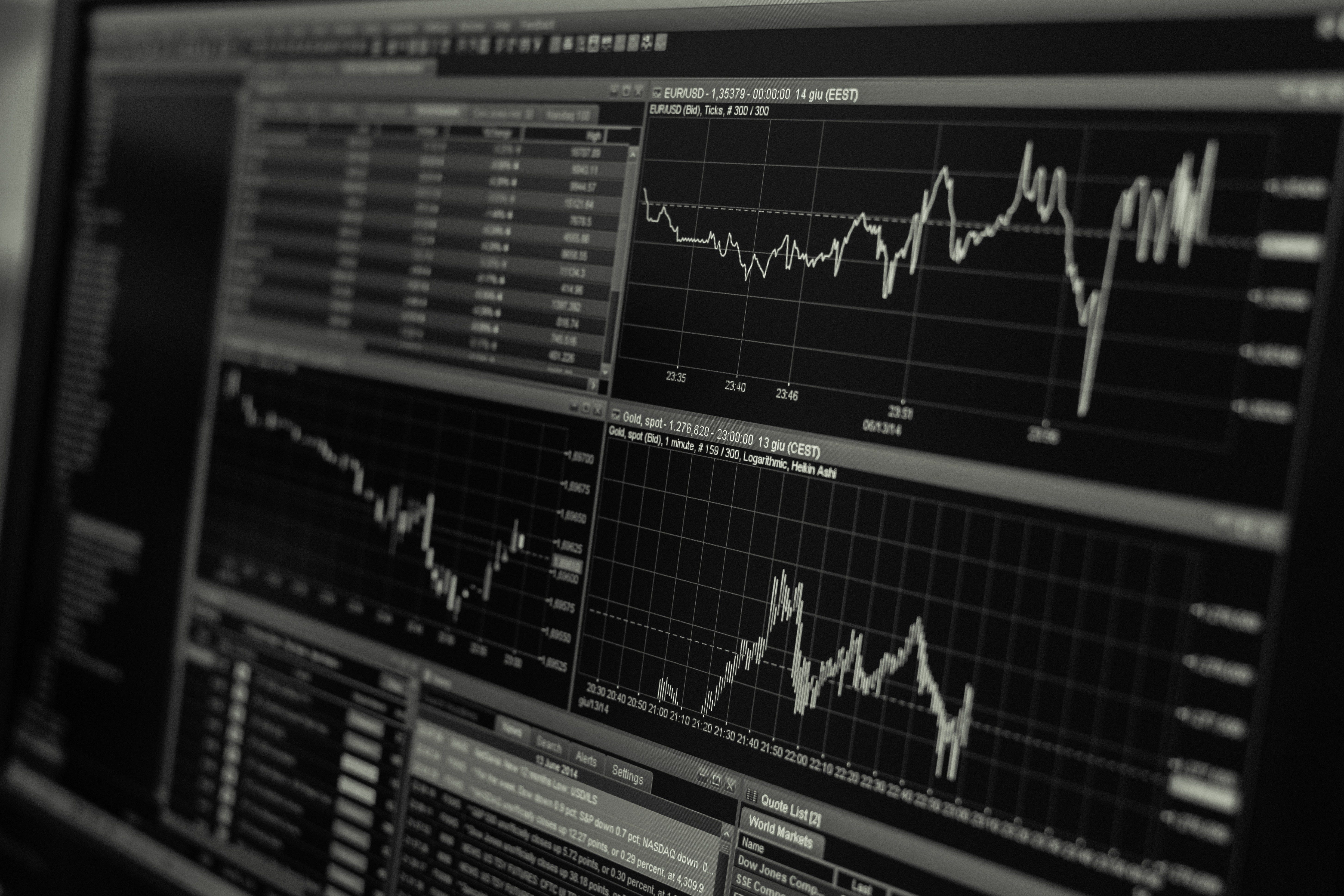An important piece of our environment is the concept of a trading session. If we were to deploy this agent into the wild, we would likely never run it for more than a couple months at a time. For this reason, we are going to limit the amount of continuous frames in `self.df` that our agent will see in a row.

In our `_reset_session` method, we are going to first reset the `current_step` to `0`. Next, we’ll set `steps_left` to a random number between `1` and `MAX_TRADING_SESSION`, which we will now define at the top of the file.

``MAX_TRADING_SESSION = 100000  # ~2 months``

Next, if we are traversing the frame serially, we will setup the entire frame to be traversed, otherwise we’ll set the `frame_start` to a random spot within `self.df`, and create a new data frame called `active_df`, which is just a slice of `self.df` from `frame_start` to `frame_start + steps_left`.

``````def _reset_session(self):
self.current_step = 0

if self.serial:
self.steps_left = len(self.df) - self.lookback_window_size - 1
self.frame_start = self.lookback_window_size
else:
self.frame_start = np.random.randint(
self.lookback_window_size, len(self.df) - self.steps_left)

self.active_df = self.df[self.frame_start -
self.lookback_window_size:self.frame_start + self.steps_left]``````

One important side effect of traversing the data frame in random slices is our agent will have much more unique data to work with when trained for long periods of time. For example, if we only ever traversed the data frame in a serial fashion (i.e. in order from `0` to `len(df)`), then we would only ever have as many unique data points as are in our data frame. Our observation space could only even take on a discrete number of states at each time step.

However, by randomly traversing slices of the data frame, we essentially manufacture more unique data points by creating more interesting combinations of account balance, trades taken, and previously seen price action for each time step in our initial data set. Let me explain with an example.

At time step 10 after resetting a serial environment, our agent will always be at the same time within the data frame, and would have had3 choices to make at each time step: buy, sell, or hold. And for each of these 3 choices, another choice would then be required: 10%, 20%, …, or 100% of the amount possible. This means our agent could experience any of (1⁰³)¹⁰ total states, for a total of 1⁰³⁰ possible unique experiences.

Now consider our randomly sliced environment. At time step 10, our agent could be at any of `len(df)` time steps within the data frame. Given the same choices to make at each time step, this means this agent could experience any of `len(df)`³⁰ possible unique states within the same 10 time steps.

While this may add quite a bit of noise to large data sets, I believe it should allow the agent to learn more from our limited amount of data. We will still traverse our test data set in serial fashion, to get a more accurate understanding of the algorithm’s usefulness on fresh, seemingly “live” data.

## Life Through The Agent’s Eyes

It can often be helpful to visual an environment’s observation space, in order to get an idea of the types of features your agent will be working with. For example, here is a visualization of our observation space rendered using OpenCV.

Each row in the image represents a row in our `observation_space`. The first 4 rows of frequency-like red lines represent the OHCL data, and the spurious orange and yellow dots directly below represent the volume. The fluctuating blue bar below that is the agent’s net worth, and the lighter blips below that represent the agent’s trades.

If you squint, you can just make out a candlestick graph, with volume bars below it and a strange morse-code like interface below that shows trade history. It looks like our agent should be able to learn sufficiently from the data in our `observation_space`, so let’s move on. Here we’ll define our `_next_observation` method, where we’ll scale the observed data from 0 to 1.

``````def _next_observation(self):
end = self.current_step + self.lookback_window_size + 1

obs = np.array([
self.active_df['Open'].values[self.current_step:end],
self.active_df['High'].values[self.current_step:end],
self.active_df['Low'].values[self.current_step:end],
self.active_df['Close'].values[self.current_step:end],
self.active_df['Volume_(BTC)'].values[self.current_step:end],
])

scaled_history = self.scaler.fit_transform(self.account_history)

obs = np.append(obs, scaled_history[:, -(self.lookback_window_size
+ 1):], axis=0)

return obs``````

### Taking Action

Now that we’ve set up our observation space, it’s time to write our `step` function, and in turn, take the agent’s prescribed action. Whenever `self.steps_left == 0` for our current trading session, we will sell any BTC we are holding and call `_reset_session()`. Otherwise, we set the `reward` to our current net worth and only set `done` to `True` if we’ve run out of money.

``````def step(self, action):
current_price = self._get_current_price() + 0.01
self._take_action(action, current_price)
self.steps_left -= 1
self.current_step += 1

if self.steps_left == 0:
self.balance += self.btc_held * current_price
self.btc_held = 0
self._reset_session()

obs = self._next_observation()
reward = self.net_worth
done = self.net_worth <= 0

return obs, reward, done, {}``````

Taking an action is as simple as getting the `current_price`, determining the specified action, and either buying or selling the specified amount of BTC. Let’s quickly write `_take_action` so we can test our environment.

``````def _take_action(self, action, current_price):
action_type = action
amount = action / 10
btc_bought = 0
btc_sold = 0
cost = 0
sales = 0

if action_type < 1:
btc_bought = self.balance / current_price * amount
cost = btc_bought * current_price * (1 + self.commission)
self.btc_held += btc_bought
self.balance -= cost

elif action_type < 2:
btc_sold = self.btc_held * amount
sales = btc_sold * current_price  * (1 - self.commission)
self.btc_held -= btc_sold
self.balance += sales``````

Finally, in the same method, we will append the trade to `self.trades` and update our net worth and account history.

``````  if btc_sold > 0 or btc_bought > 0:
'step': self.frame_start+self.current_step,
'amount': btc_sold if btc_sold > 0 else btc_bought,
'total': sales if btc_sold > 0 else cost,
'type': "sell" if btc_sold > 0 else "buy"
})

self.net_worth = self.balance + self.btc_held * current_price
self.account_history = np.append(self.account_history, [
[self.net_worth],
[btc_bought],
[cost],
[btc_sold],
[sales]
], axis=1)``````

Our agents can now initiate a new environment, step through that environment, and take actions that affect the environment. It’s time to watch them trade.

Our `render` method could be something as simple as calling `print(self.net_worth)`, but that’s no fun. Instead we are going to plot a simple candlestick chart of the pricing data with volume bars and a separate plot for our net worth.

We are going to take the code in `StockTradingGraph.py` from the last article I wrote, and re-purposing it to render our Bitcoin environment. You can grab the code from my GitHub.

The first change we are going to make is to update `self.df['Date']` everywhere to `self.df['Timestamp']`, and remove all calls to `date2num` as our dates already come in unix timestamp format. Next, in our `render` method we are going to update our date labels to print human-readable dates, instead of numbers.

``from datetime import datetime``

First, import the `datetime` library, then we’ll use the `utcfromtimestamp` method to get a UTC string from each timestamp and `strftime` to format the string in `Y-m-d H:M` format.

``````date_labels = np.array([datetime.utcfromtimestamp(x).strftime(
'%Y-%m-%d %H:%M') for x in self.df['Timestamp'].values[step_range]])``````

Finally, we change `self.df['Volume']` to `self.df['Volume_(BTC)']` to match our data set, and we’re good to go. Back in our `BitcoinTradingEnv`, we can now write our `render` method to display the graph.

``````def render(self, mode='human', **kwargs):
if mode == 'human':
if self.viewer == None:
kwargs.get('title', None))

self.viewer.render(self.frame_start + self.current_step,
self.net_worth,
window_size=self.lookback_window_size)``````

And voila! We can now watch our agents trade Bitcoin.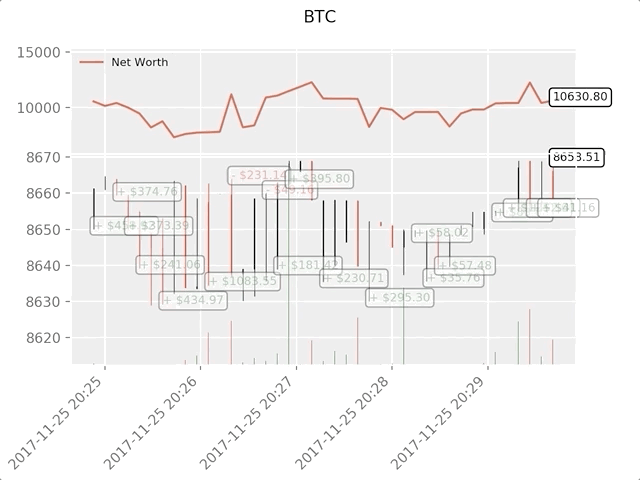Matplotlib visualization of our agent trading Bitcoin

The green ghosted tags represent buys of BTC and the red ghosted tags represent sells. The white tag on the top right is the agent’s current net worth and the bottom right tag is the current price of Bitcoin. Simple, yet elegant. Now, it’s time to train our agent and see how much money we can make!

### Training Time

One of the criticisms I received on my first article was the lack of cross-validation, or splitting the data into a training set and test set. The purpose of doing this is to test the accuracy of your final model on fresh data it has never seen before. While this was not a concern of that article, it definitely is here. Since we are using time series data, we don’t have many options when it comes to cross-validation.

For example, one common form of cross validation is called k-fold validation, in which you split the data into k equal groups and one by one single out a group as the test group and use the rest of the data as the training group. However time series data is highly time dependent, meaning later data is highly dependent on previous data. So k-fold won’t work, because our agent will learn from future data before having to trade it, an unfair advantage.

This same flaw applies to most other cross-validation strategies when applied to time series data. So we are left with simply taking a slice of the full data frame to use as the training set from the beginning of the frame up to some arbitrary index, and using the rest of the data as the test set.

``````slice_point = int(len(df) - 100000)
train_df = df[:slice_point]
test_df = df[slice_point:]``````

Next, since our environment is only set up to handle a single data frame, we will create two environments, one for the training data and one for the test data.

``````train_env = DummyVecEnv([lambda: BitcoinTradingEnv(train_df,
commission=0, serial=False)])
commission=0, serial=True)])``````

Now, training our model is as simple as creating an agent with our environment and calling `model.learn`.

``````model = PPO2(MlpPolicy,
train_env,
verbose=1,
tensorboard_log="./tensorboard/")
model.learn(total_timesteps=50000)``````

Here, we are using tensorboard so we can easily visualize our tensorflow graph and view some quantitative metrics about our agents. For example, here is a graph of the discounted rewards of many agents over 200,000 time steps: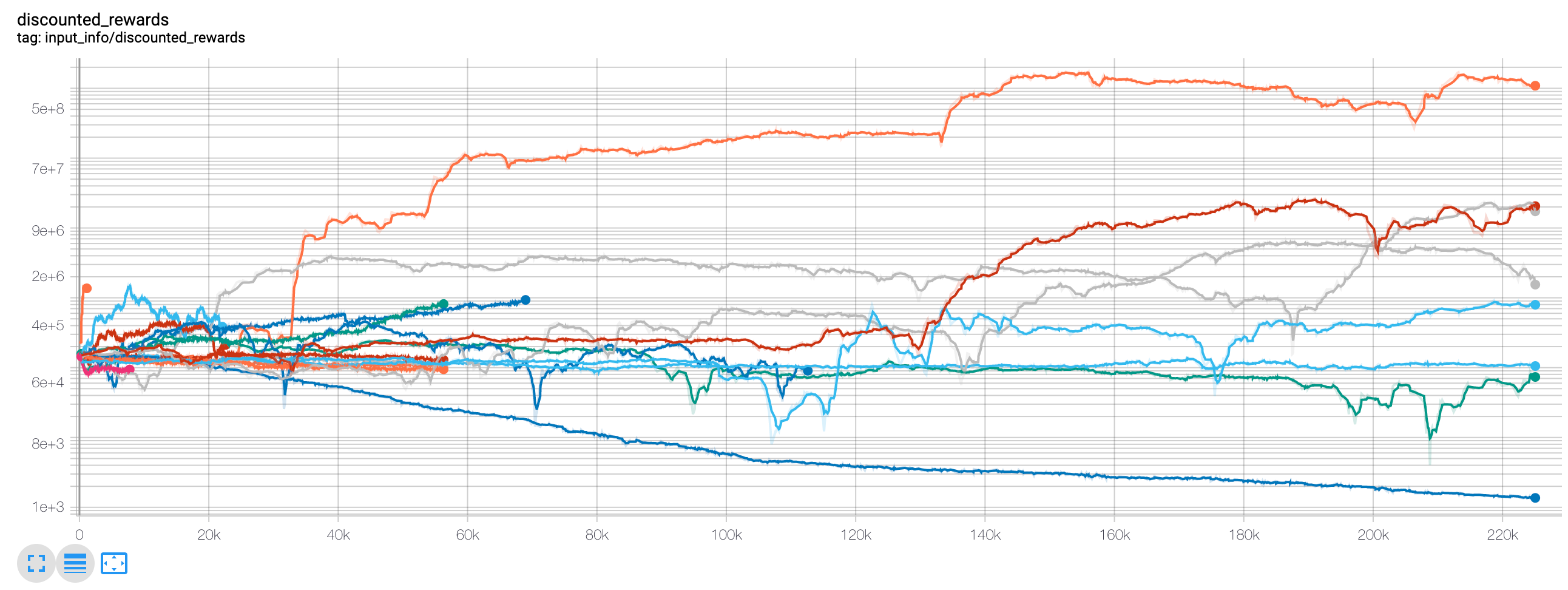Wow, it looks like our agents are extremely profitable! Our best agent was even capable of 1000x’ing his balance over the course of 200,000 steps, and the rest averaged at least a 30x increase!

It was at this point that I realized there was a bug in the environment… Here is the new rewards graph, after fixing that bug: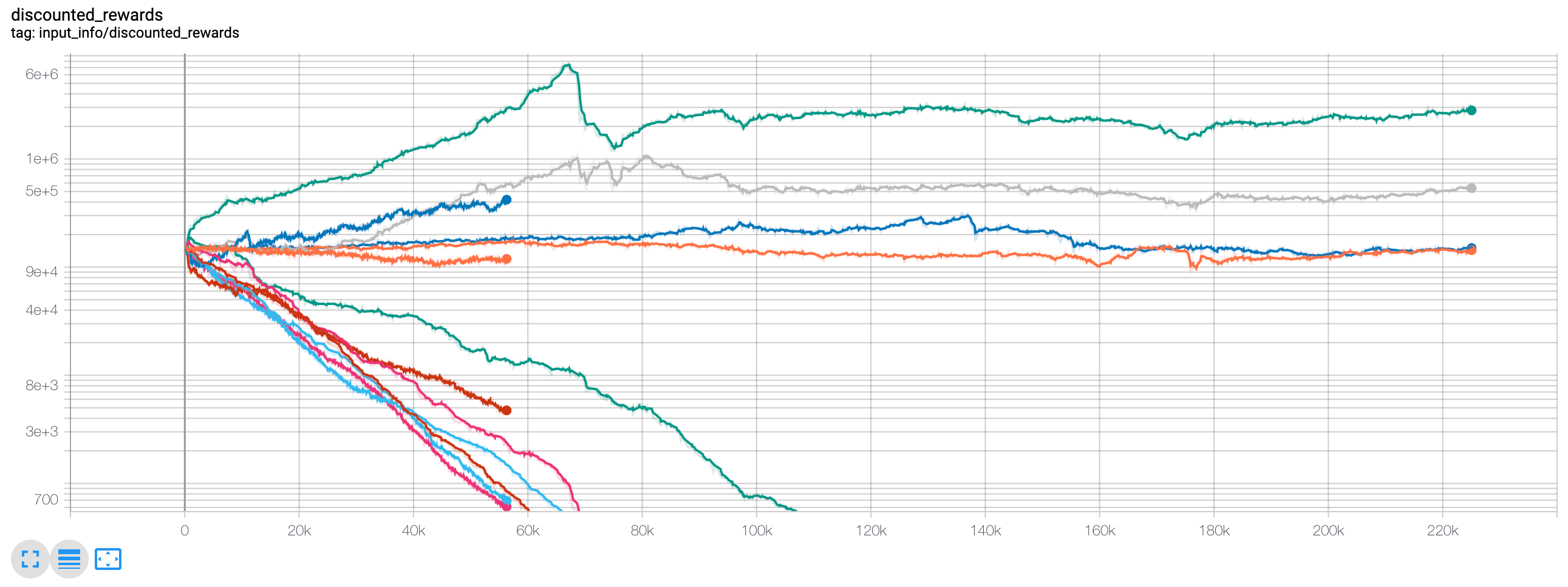As you can see, a couple of our agents did well, and the rest traded themselves into bankruptcy. However, the agents that did well were able to 10x and even 60x their initial balance, at best. I must admit, all of the profitable agents were trained and tested in an environment without commissions, so it is still entirely unrealistic for our agent’s to make any real money. But we’re getting somewhere!

Let’s test our agents on the test environment (with fresh data they’ve never seen before), to see how well they’ve learned to trade Bitcoin.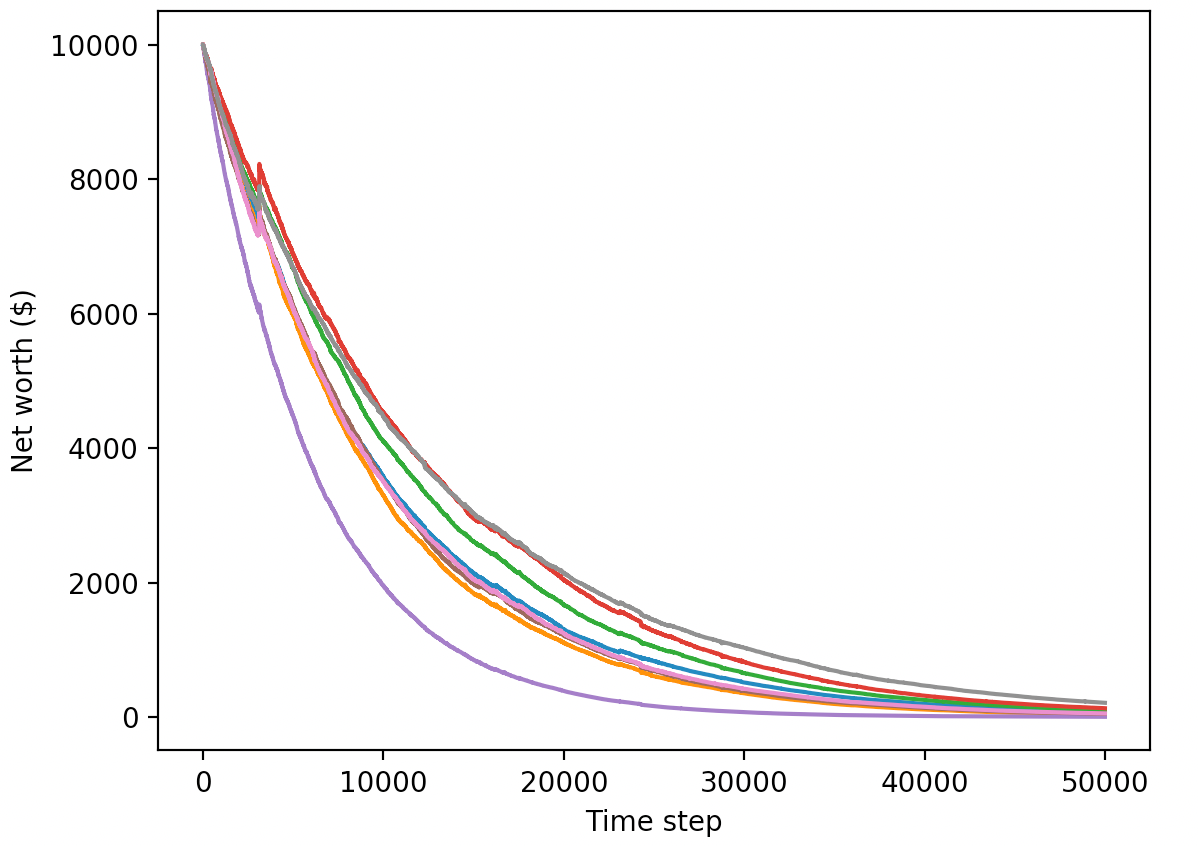Our trained agents race to bankruptcy when trading on fresh, test data

Clearly, we’ve still got quite a bit of work to do. By simply switching our model to use stable-baseline’s A2C, instead of the current PPO2 agent, we can greatly improve our performance on this data set. Finally, we can update our reward function slightly, as per Sean O’Gorman’s advice, so that we reward increases in net worth, not just achieving a high net worth and staying there.

``reward = self.net_worth - prev_net_worth``

These two changes alone greatly improve the performance on the test data set, and as you can see below, we are finally able to achieve profitability on fresh data that wasn’t in the training set.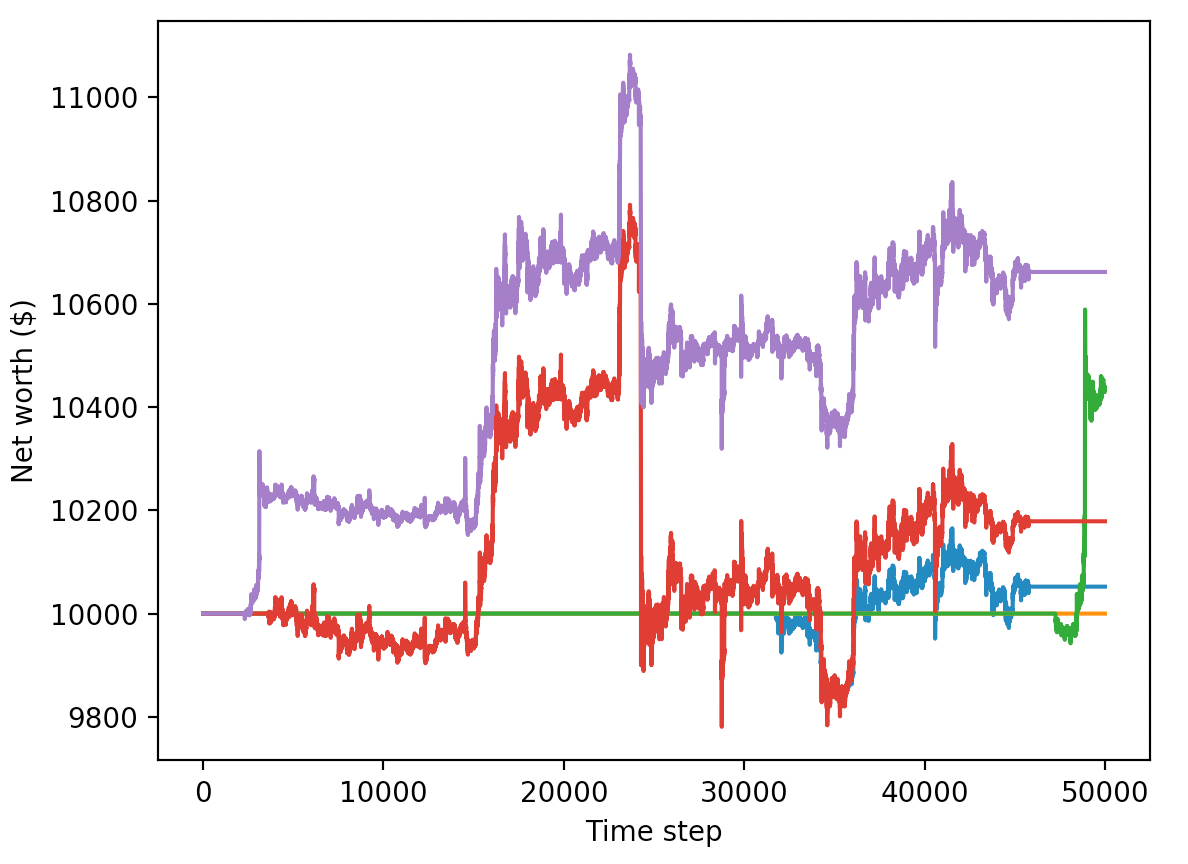However, we can do much better. In order for us to improve these results, we are going to need to optimize our hyper-parameters and train our agents for much longer. Time to break out the GPU and get to work!

However, this article is already a bit long and we’ve still got quite a bit of detail to go over, so we are going to take a break here. In my next article, we will use Bayesian optimization to zone in on the best hyper-parameters for our problem space, and improve the agent’s model to achieve highly profitable trading strategies.

# Conclusion

In this article, we set out to create a profitable Bitcoin trading agent from scratch, using deep reinforcement learning. We were able to accomplish the following:

1. Created a Bitcoin trading environment from scratch using OpenAI’s gym.
2. Built a visualization of that environment using Matplotlib.
3. Trained and tested our agents using simple cross-validation.
4. Tuned our agent slightly to achieve profitability.

While our trading agent isn’t quite as profitable as we’d hoped, it is definitely getting somewhere. Next time, we will improve on these algorithms through advanced feature engineering and Bayesian optimization to make sure our agents can consistently beat the market.

Thanks for reading! As always, all of the code for this tutorial can be found on _ GitHub. Leave a comment below if you have any questions or feedback,

## PyTorch for Deep Learning | Data Science | Machine Learning | Python

PyTorch for Deep Learning | Data Science | Machine Learning | Python. PyTorch is a library in Python which provides tools to build deep learning models. What python does for programming PyTorch does for deep learning. Python is a very flexible language for programming and just like python, the PyTorch library provides flexible tools for deep learning.

## Deep Learning Tutorial with Python | Machine Learning with Neural Networks

In this video, Deep Learning Tutorial with Python | Machine Learning with Neural Networks Explained, Frank Kane helps de-mystify the world of deep learning and artificial neural networks with Python!

## PyTorch for Deep Learning | Data Science | Machine Learning | Python

PyTorch is a library in Python which provides tools to build deep learning models. What python does for programming PyTorch does for deep learning.

## Machine Learning, Data Science and Deep Learning with Python

Complete hands-on Machine Learning tutorial with Data Science, Tensorflow, Artificial Intelligence, and Neural Networks. Introducing Tensorflow, Using Tensorflow, Introducing Keras, Using Keras, Convolutional Neural Networks (CNNs), Recurrent Neural Networks (RNNs), Learning Deep Learning, Machine Learning with Neural Networks, Deep Learning Tutorial with Python

## How To Plot A Decision Boundary For Machine Learning Algorithms in Python

How To Plot A Decision Boundary For Machine Learning Algorithms in Python, you will discover how to plot a decision surface for a classification machine learning algorithm.